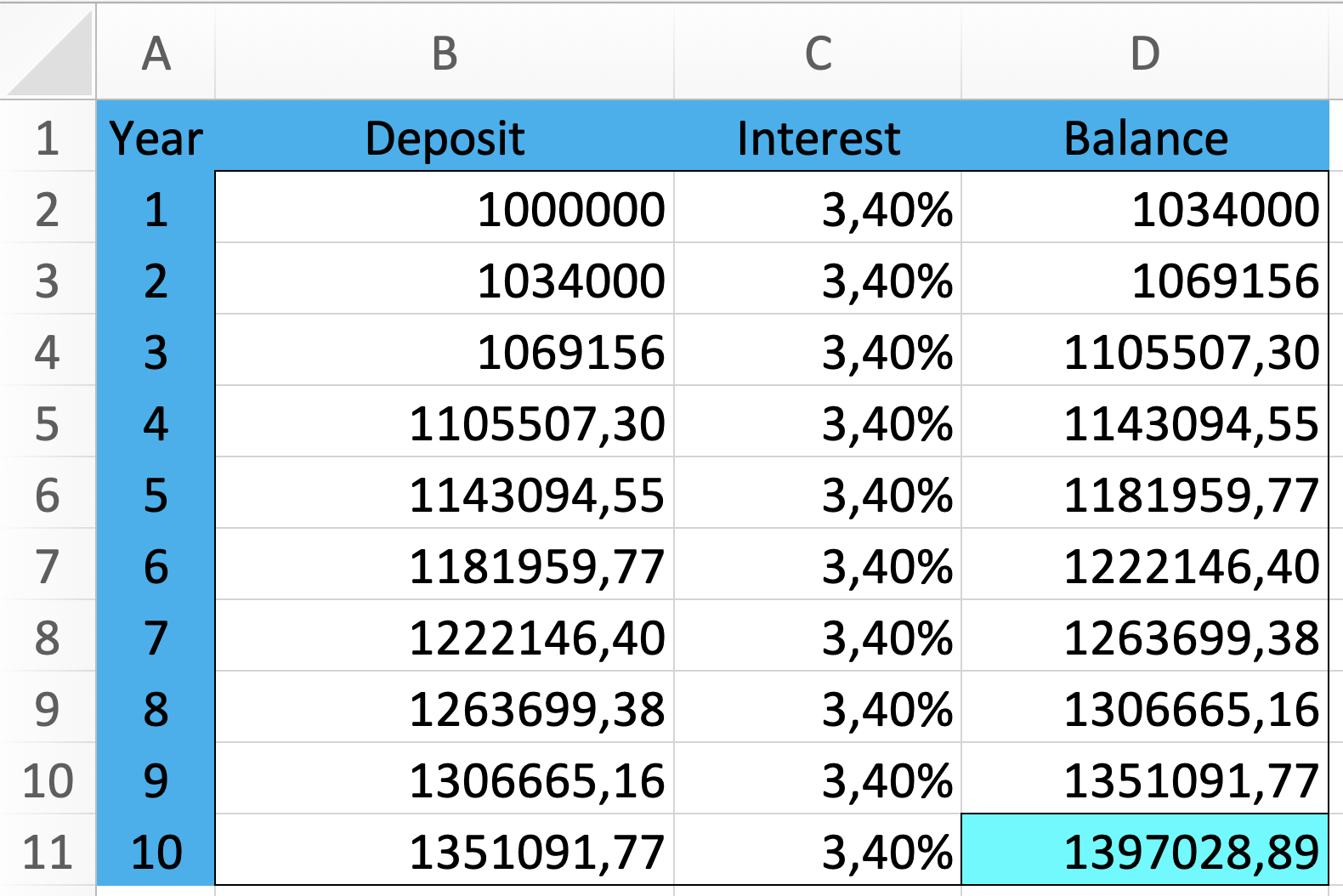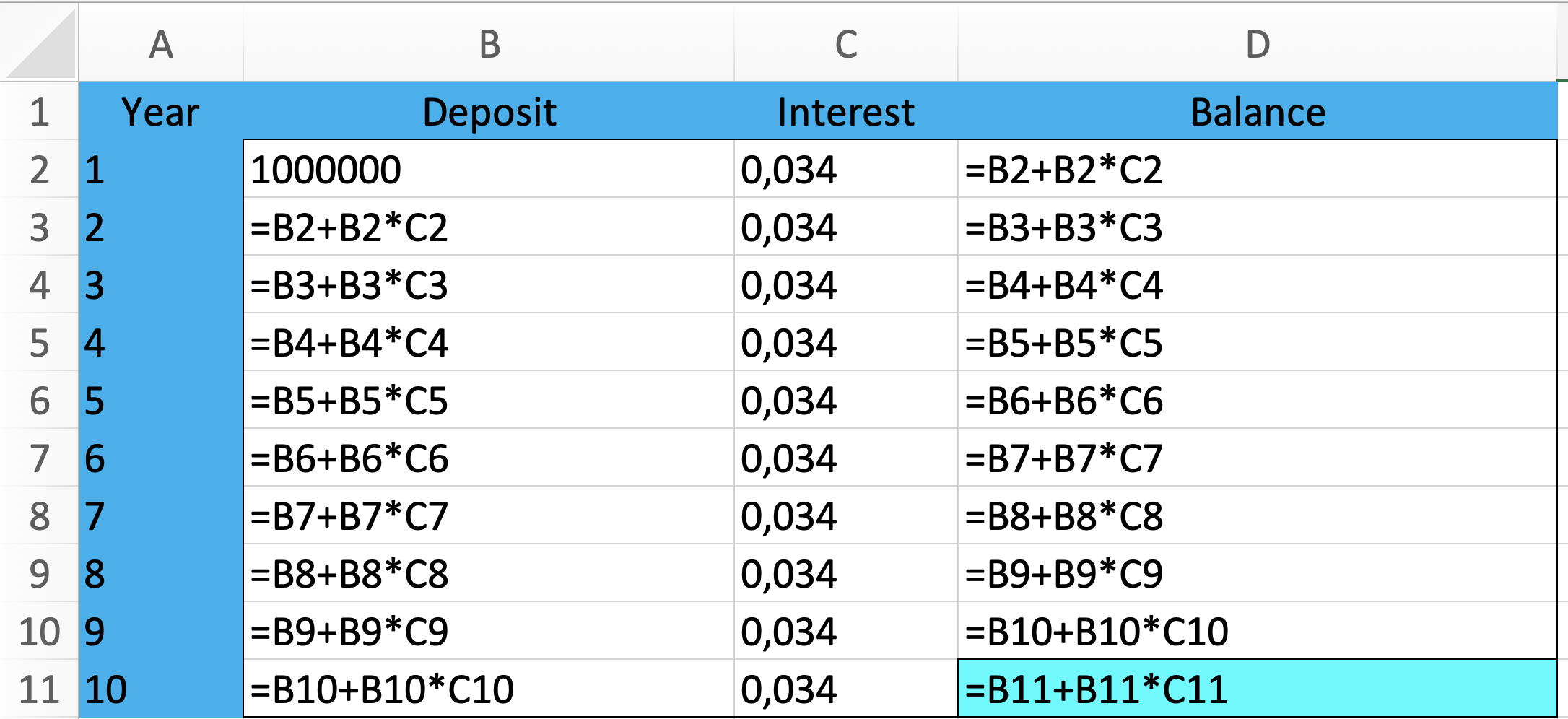Math Topics

# How to Compute Compound Interest on Savings

When you save money in a bank, the money often stays in your account for many years. The money you put in your account is called a deposit. You receive interest on this money. All of your deposits, plus the interest you’ve earned, comprise your account balance.

When you leave the total balance of your savings in your account for several years, you don’t only receive interest on the amount you deposited, you also receive interest on top of the interest you already earned. This interest on earned interest is called compound interest, and must be taken into account when you calculate. How much money you’ll have in your account after $n$ years follows this pattern:

Formula

### Savingsovertime

Interest $i$ will grow your balance in the following way:

Balance after 1 year:

 $\text{deposit}+\text{deposit}\cdot i\phantom{\rule{0.33em}{0ex}}\text{%}$

Balance after 2 years:

balance after 3 years:

balance after n years:

This step-by-step illustration was included to help you see what’s happening. But multi-year interest is often calculated using `Excel`, so we’ll add an example from `Excel` as well.

Example 1

Uncle Scrooge has earned a lot of money through his many different ventures. The first million he made, he put in a savings account at the bank. Scrooge left that money in the bank for 10 years before he withdrew it. The bank gave him an interest rate of $\text{}3.4\text{}\phantom{\rule{0.17em}{0ex}}\text{%}$. How much money did he have in the bank after 10 years?

The top table shows the calculated numbers. The bottom table shows the formulas to use in `Excel`.Scrooge had \$$1\phantom{\rule{0.17em}{0ex}}397\phantom{\rule{0.17em}{0ex}}028.89$ in the bank after $10$ years. That means he had earned \$$397\phantom{\rule{0.17em}{0ex}}028.89$ in interest.